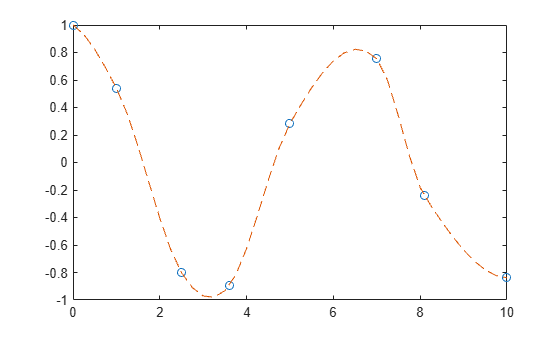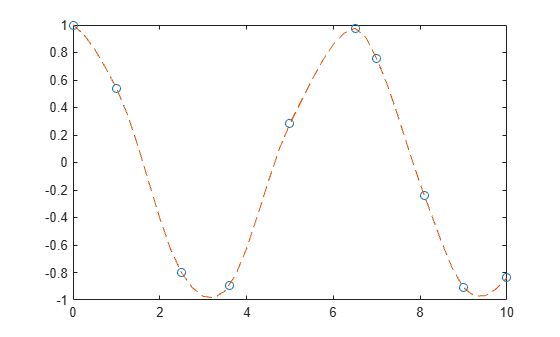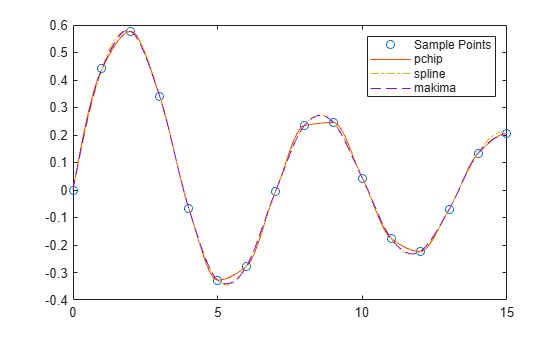# makima

Modified Akima piecewise cubic Hermite interpolation

Since R2019b

## Syntax

``yq = makima(x,y,xq)``
``pp = makima(x,y)``

## Description

example

````yq = makima(x,y,xq)` performs Modified Akima Interpolation using the values `y` at sample points `x` to find interpolated values `yq` at the query points `xq`.```

example

````pp = makima(x,y)` returns a piecewise polynomial structure for use with `ppval` and the spline utility `unmkpp`.```

## Examples

collapse all

Use `makima` to interpolate a cosine curve over unevenly spaced sample points.

```x = [0 1 2.5 3.6 5 7 8.1 10]; y = cos(x); xq = 0:.25:10; yq = makima(x,y,xq); plot(x,y,'o',xq,yq,'--')```With oscillatory functions, the Akima algorithm flattens the curve near local extrema. To compensate for this flattening, you can add more sample points near the local extrema.

Add sample points near $\mathit{x}=6.5$ and $\mathit{x}=9$ and replot the interpolation.

```x = [0 1 2.5 3.6 5 6.5 7 8.1 9 10]; y = cos(x); xq = 0:.25:10; yq = makima(x,y,xq); plot(x,y,'o',xq,yq,'--')```Compare the interpolation results produced by `spline`, `pchip`, and `makima` for two different data sets. These functions all perform different forms of piecewise cubic Hermite interpolation. Each function differs in how it computes the slopes of the interpolant, leading to different behaviors when the underlying data has flat areas or undulations.

Compare the interpolation results on sample data that connects flat regions. Create vectors of `x` values, function values at those points `y`, and query points `xq`. Compute interpolations at the query points using `spline`, `pchip`, and `makima`. Plot the interpolated function values at the query points for comparison.

```x = -3:3; y = [-1 -1 -1 0 1 1 1]; xq1 = -3:.01:3; p = pchip(x,y,xq1); s = spline(x,y,xq1); m = makima(x,y,xq1); plot(x,y,'o',xq1,p,'-',xq1,s,'-.',xq1,m,'--') legend('Sample Points','pchip','spline','makima','Location','SouthEast')```In this case, `pchip` and `makima` have similar behavior in that they avoid overshoots and can accurately connect the flat regions.

Perform a second comparison using an oscillatory sample function.

```x = 0:15; y = besselj(1,x); xq2 = 0:0.01:15; p = pchip(x,y,xq2); s = spline(x,y,xq2); m = makima(x,y,xq2); plot(x,y,'o',xq2,p,'-',xq2,s,'-.',xq2,m,'--') legend('Sample Points','pchip','spline','makima')```When the underlying function is oscillatory, `spline` and `makima` capture the movement between points better than `pchip`, which is aggressively flattened near local extrema.

Create vectors for the sample points `x` and values at those points `y`. Use `makima` to construct a piecewise polynomial structure for the data.

```x = -5:5; y = [1 1 1 0 0 1 1 2 2 2 2]; pp = makima(x,y)```
```pp = struct with fields: form: 'pp' breaks: [-5 -4 -3 -2 -1 0 1 2 3 4 5] coefs: [10x4 double] pieces: 10 order: 4 dim: 1 ```

The structure contains the information for 10 polynomials of order 4 that span the data. `pp.coefs(i,:)` contains the coefficients for the polynomial that is valid in the region defined by the breakpoints `[breaks(i) breaks(i+1)]`.

Use the structure with `ppval` to evaluate the interpolation at several query points, and then plot the results. In regions with three or more constant points, the Akima algorithm connects the points with a straight line.

```xq = -5:0.2:5; m = ppval(pp,xq); plot(x,y,'o',xq,m,'-.') ylim([-0.2 2.2])```## Input Arguments

collapse all

Sample points, specified as a vector. The vector `x` specifies the points at which the data `y` is given. The elements of `x` must be unique.

Data Types: `single` | `double`

Function values at sample points, specified as a numeric vector, matrix, or array. `x` and `y` must have the same length.

If `y` is a matrix or array, then the values in the last dimension, `y(:,...,:,j)`, are taken as the values to match with `x`. In that case, the last dimension of `y` must be the same length as `x`.

Data Types: `single` | `double`

Query points, specified as a scalar, vector, matrix, or array. The points specified in `xq` are the x-coordinates for the interpolated function values `yq` computed by `makima`.

Data Types: `single` | `double`

## Output Arguments

collapse all

Interpolated values at query points, returned as a scalar, vector, matrix, or array. The size of `yq` is related to the sizes of `y` and `xq`:

• If `y` is a vector, then `yq` has the same size as `xq`.

• If `y` is an array of size `Ny = size(y)`, then these conditions apply:

• If `xq` is a scalar or vector, then `size(yq)` returns ```[Ny(1:end-1) length(xq)]```.

• If `xq` is an array, then `size(yq)` returns `[Ny(1:end-1) size(xq)]`.

Piecewise polynomial, returned as a structure. Use this structure with the `ppval` function to evaluate the interpolating polynomials at one or more query points. The structure has these fields.

FieldDescription
`form`

`'pp'` for piecewise polynomial

`breaks`

Vector of length `L+1` with strictly increasing elements that represent the start and end of each of` L` intervals

`coefs`

`L`-by-`k` matrix with each row `coefs(i,:)` containing the local coefficients of an order `k` polynomial on the `i`th interval, `[breaks(i),breaks(i+1)]`

`pieces`

Number of pieces, `L`

`order`

Order of polynomials

`dim`

Dimensionality of target

Because the polynomial coefficients in `coefs` are local coefficients for each interval, you must subtract the lower endpoint of the corresponding knot interval to use the coefficients in a conventional polynomial equation. In other words, for the coefficients `[a,b,c,d]` on the interval `[x1,x2]`, the corresponding polynomial is

`$f\left(x\right)=a{\left(x-{x}_{1}\right)}^{3}+b{\left(x-{x}_{1}\right)}^{2}+c\left(x-{x}_{1}\right)+d\text{\hspace{0.17em}}.$`

collapse all

### Modified Akima Interpolation

The Akima algorithm for one-dimensional interpolation, described in  and , performs cubic interpolation to produce piecewise polynomials with continuous first-order derivatives (C1). The algorithm avoids excessive local undulations.

If ${\delta }_{i}=\frac{{v}_{i+1}-{v}_{i}}{{x}_{i+1}-{x}_{i}}$ is the slope on interval $\left[{x}_{i}\text{\hspace{0.17em}}\text{\hspace{0.17em}}{x}_{i+1}\right)$, then the value of the derivative ${d}_{i}$ at the sample point ${x}_{i}$ is a weighted average of nearby slopes:

`${d}_{i}=\frac{{w}_{1}}{{w}_{1}+{w}_{2}}{\delta }_{i-1}+\frac{{w}_{2}}{{w}_{1}+{w}_{2}}{\delta }_{i}.$`

In Akima's original formula, the weights are:

`$\begin{array}{l}{w}_{1}=|{\delta }_{i+1}-{\delta }_{i}|,\\ {w}_{2}=|{\delta }_{i-1}-{\delta }_{i-2}|.\end{array}$`

The original Akima algorithm gives equal weight to the points on both sides, evenly dividing an undulation.

When two flat regions with different slopes meet, the modification made to the original Akima algorithm gives more weight to the side where the slope is closer to zero. This modification gives priority to the side that is closer to horizontal, which is more intuitive and avoids overshoot. In particular, whenever there are three or more consecutive collinear points, the algorithm connects them with a straight line and thus avoids an overshoot.

The weights used in the modified Akima algorithm are:

`$\begin{array}{l}{w}_{1}=|{\delta }_{i+1}-{\delta }_{i}|+\frac{|{\delta }_{i+1}+{\delta }_{i}|}{2},\\ {w}_{2}=|{\delta }_{i-1}-{\delta }_{i-2}|+\frac{|{\delta }_{i-1}+{\delta }_{i-2}|}{2}.\end{array}$`

Compared to the `spline` algorithm, the Akima algorithm produces fewer undulations and is better suited to deal with quick changes between flat regions. Compared to the `pchip` algorithm, the Akima algorithm is not as aggressively flattened and is therefore still able to deal with oscillatory data.

 Akima, Hiroshi. "A new method of interpolation and smooth curve fitting based on local procedures." Journal of the ACM (JACM) , 17.4, 1970, pp. 589–602.

 Akima, Hiroshi. "A method of bivariate interpolation and smooth surface fitting based on local procedures." Communications of the ACM , 17.1, 1974, pp. 18–20.